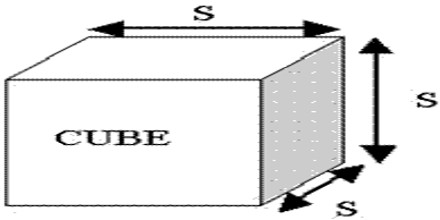Volume of a Cube

A cube is a three-dimensional shape that has equal width, height, and length measurements. A cube has six square faces, all of which have sides of equal length and all of which meet at right angles.

The volume of cube is (length of side)3

Finding the volume of a cube is a snap – generally, all that’s needed is to multiply the cube’s length × width × height.Example: Since a cube’s sides are all equal in length, another way of thinking of a cube’s volume is s3, where s is the length of one of the cube’s sides. Find the volume if the length of one side is 2 cm.

VCube = 23

Vcube = 2 × 2 × 2

Vcube = 8 cm3

Units

Remember that the length of an edge and the volume will be in similar units. So if the edge length is in miles, then the volume will be in cubic miles, and so on.